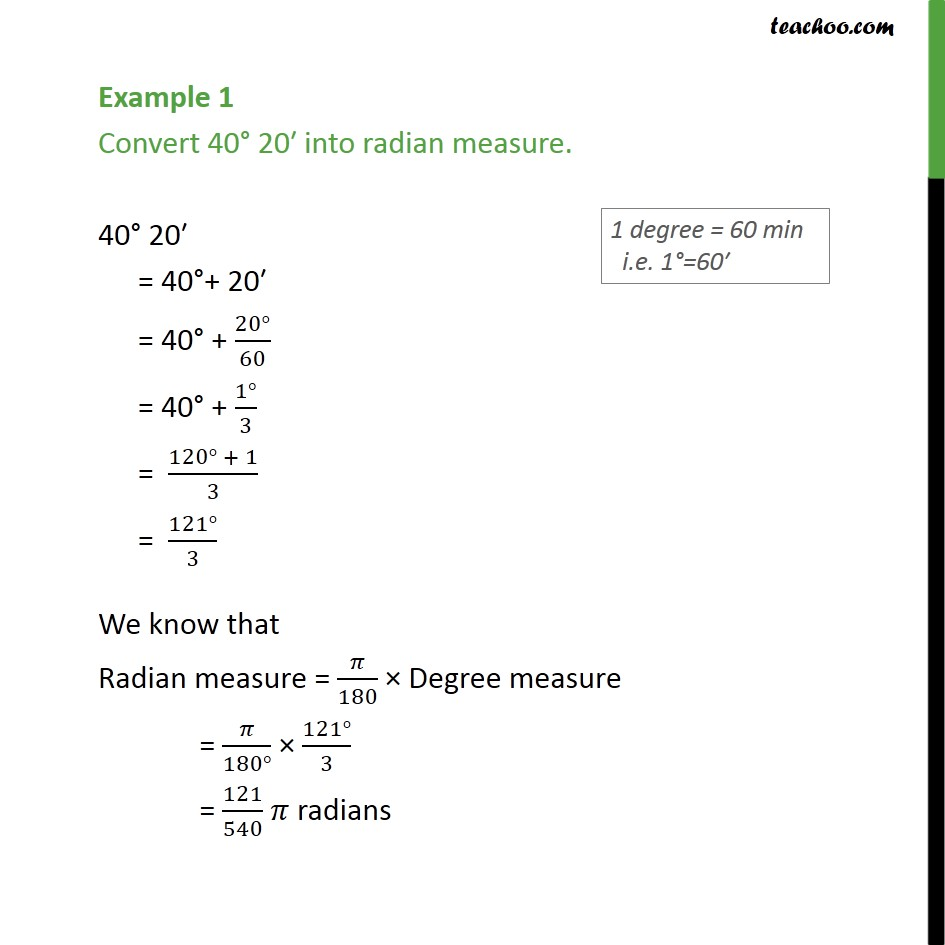1. Chapter 3 Class 11 Trigonometric Functions (Term 2)
2. Concept wise

Transcript

Example 1 Convert 40 20 into radian measure. 40 20 = 40 + 20 = 40 + (20 )/60 = 40 + (1 )/3 = (120 + 1)/3 = (121 )/3 We know that Radian measure = /180 Degree measure = /(180 ) (121 )/3 = 121/540 radians